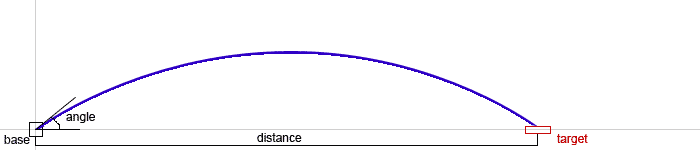DWITE Online Computer Programming Contest
January 2008
Problem 1
Curve-shot

In a typical tactical shooting game, trajectory shots are fired from base to target. Given an angle of the aim and power of the shot, we are interested in the distance the projective will travel.Standard rules of physics apply. Shot is fired from the center of the base, at the initial velocity specified, in meters per second. The angle determines horizontal and vertical components of the velocity and is supplied in degrees. The target is on the same level as the base. Gravity supplies constant downward acceleration of 9.81 meters per second^2. There is no wind, or any friction.

The input file DATA1.txt will contain five lines, each in angle power format. Angle in degrees, an integer, 0 <= d <= 90. Power in meter per second, an integer, 0 <= p <= 100.

The output file OUT1.txt will contain five lines, each stating the expected distance, rounded to the nearest integer.

Sample Input:
```10 100
25 100
40 100
60 100
85 100
```
Sample Output:
```349
781
1004
883
177
```